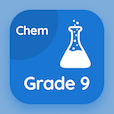Class 10 Online Courses

Grade 10 Math MCQs PDF - Topics

# Recognize of Operations on Sets MCQ Quiz Online

Learn Recognize of operations on sets Multiple Choice Questions (MCQ) to practice recognize of operations on sets quiz answers PDF to study online 10th grade math course. Sets and Functions Multiple Choice Questions and Answers (MCQs), Recognize of Operations on Sets quiz questions PDF for online schools. "Recognize of Operations on Sets MCQ" PDF eBook: sets, binary relation, recognize of operations on sets test prep for online high school courses.

"If 2 sets A and B are given, then the set consisting of all the elements which are either in A or in B or in both is called" Multiple Choice Questions (MCQ) on recognize of operations on sets with choices intersection of a and b, union of a and b, complement of a, and complement of b for online schools. Study sets and functions quiz questions for online certificate programs for online school and college.

## MCQs on Recognize of Operations on Sets

MCQ: If 2 sets A and B are given, then the set consisting of all the elements which are either in A or in B or in both is called

Intersection of A and B
union of A and B
Complement of A
Complement of B

MCQ: If U = {1,2,3,4,5} and A = {2,4} then A' should be

{2,4,5}
{2,4}
{1,2,3,4,5}
{1,3,5}

MCQ: The union of set A and B is denoted by

A + B
A - B
A∩B
A∪B

MCQ: If X = {1, 2, 3, 4, 5} and Y = {2, 4, 5, 6, 8} then Y - X equals to

{4, 5}
{1, 6}
{6, 8}
{1, 3}

MCQ: If A = {1, 2, 3, 4} and B = {4, 5, 6, 7} then A∪B should be

{2, 3, 4, 5, 6, 7}
{1, 2, 3, 4, 5, 6, 7}
{4}
{1, 2, 3, 5, 6, 7}

### More Topics from Grade 10 Math Course